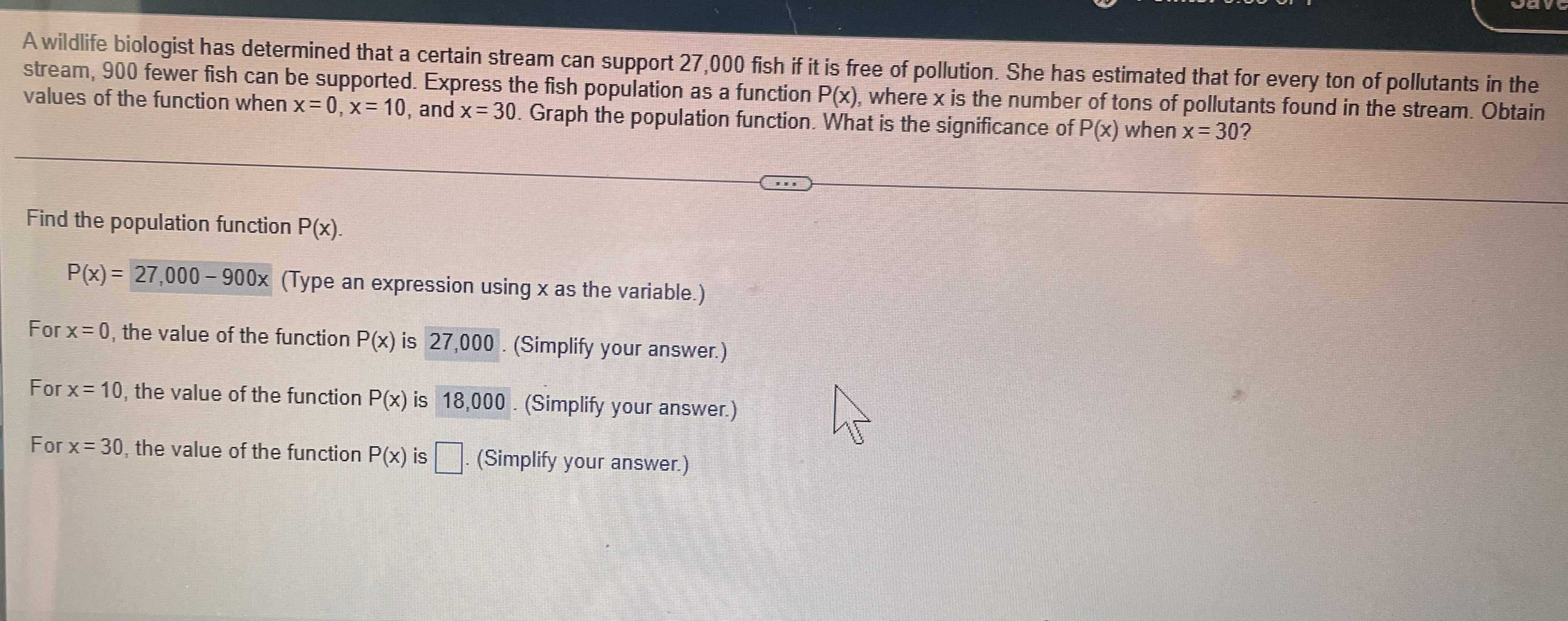### Still have math questions?

Algebra
QuestionA wildlife biologist has determined that a certain stream can support $$27,000$$ fish if it is free of pollution. She has estimated that for every ton of pollutants in the stream, $$900$$ fewer fish can be supported. Express the fish population as a function $$P ( x )$$ , where $$x$$ is the number of tons of pollutants found in the stream. Obtain values of the function when $$x = 0 , x = 10$$ , and $$x = 30$$ . Graph the population function. What is the significance of $$P ( x )$$ when $$x = 30$$ ?

Find the population function $$P ( x )$$ .

$$P ( x ) = 27,000 - 900 x$$

(Type an expression using $$x$$ as the variable.)

For $$x = 0$$ , the value of the function $$P ( x )$$ is $$27,000$$ . (Simplify your answer.)

For $$x = 10$$ , the value of the function $$P ( x )$$ is $$18,000$$ . (Simplify your answer.)

For $$x = 30$$ , the value of the function $$P ( x )$$ is $$\square$$ . (Simplify your answer.)